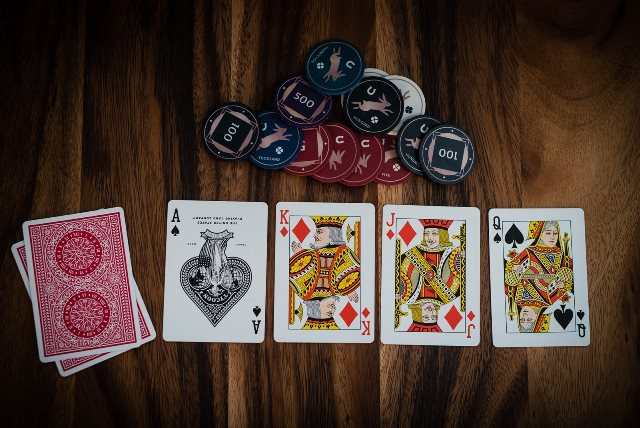# Conditional Probability – Understanding Conditional Probability with Examples

Probability is a field of study that deals with the likelihood of events occurring. One of the fundamental concepts in this field is “conditional probability.” At its core, conditional probability helps us understand the probability of an event occurring given that another event has already occurred. This concept is pivotal in many real-world scenarios, from medical diagnoses to financial forecasting.In this Blog post we will learn:
1. Definition of Conditional Probability
2. Example 1: Drawing Cards
3. Example 2: Health Diagnosis
4. Example 3: Weather and Traffic
5. Conclusion

## 1. Definition of Conditional Probability

Mathematically, the conditional probability of Event $A$ given that Event $B$ has occurred is represented as:

$P(A|B) = \frac{P(A \cap B)}{P(B)}$

Where:
– $P(A|B)$ is the conditional probability of $A$ given $B$.
– $P(A \cap B)$ is the probability of both $A$ and $B$ occurring.
– $P(B)$ is the probability of $B$ occurring.

## 2. Example 1: Drawing Cards

Consider a deck of 52 playing cards. If you draw a card, the probability that it’s a King (Event $A$) is 4/52 or 1/13.

Now, suppose you know the card you’ve drawn is a face card (Event $B$). Given this information, the probability it’s a King has changed. There are 12 face cards (Kings, Queens, Jacks) in total. Of those, 4 are Kings. Therefore, the conditional probability of drawing a King given that you’ve drawn a face card is:

$P(A|B) = \frac{4}{12} = \frac{1}{3}$

## 3. Example 2: Health Diagnosis

Suppose in a city, 1% of people have a particular disease (Event $A$). There’s a test for the disease, but it’s not perfect. If you have the disease, the test will correctly identify it 99% of the time. However, if you don’t have the disease, the test will still say you do 2% of the time.

If someone tests positive (Event $B$), what’s the probability they actually have the disease?

Using Bayes’ theorem:

$P(A|B) = \frac{P(B|A) \times P(A)}{P(B)}$

Where:
– $P(B|A)$ is the probability of testing positive given you have the disease, which is 0.99.
– $P(A)$ is the probability of having the disease, which is 0.01.
– $P(B)$ is the total probability of testing positive, which includes both true positives and false positives.

$P(B) = P(A) \times P(B|A) + P(not A) \times P(B|not A) = 0.01 \times 0.99 + 0.99 \times 0.02 = 0.0297$

Substituting these values in:

$P(A|B) = \frac{0.99 \times 0.01}{0.0297} \approx 0.332$

Thus, even if someone tests positive, there’s only a 33.2% chance they actually have the disease.

## 4. Example 3: Weather and Traffic

Suppose in a city, on any given day, there’s a 20% chance of rain (Event $A$). If it rains, there’s an 80% chance of traffic jams (Event $B$). On days without rain, there’s a 30% chance of traffic jams due to other factors.

If there’s a traffic jam, what’s the probability it rained?

$P(A|B) = \frac{P(B|A) \times P(A)}{P(B)}$

Where:
– $P(B|A)$ is the probability of a traffic jam given it rained, which is 0.80.
– $P(A)$ is the probability of rain, which is 0.20.
– $P(B)$ is the total probability of a traffic jam.

$P(B) = P(A) \times P(B|A) + P(not A) \times P(B|not A) = 0.20 \times 0.80 + 0.80 \times 0.30 = 0.44$

Substituting these values in:

$P(A|B) = \frac{0.80 \times 0.20}{0.44} \approx 0.363$

Therefore, given there’s a traffic jam, there’s a 36.3% chance it rained.

## 5. Conclusion

Conditional probability allows us to refine our predictions based on new information. It’s essential in various fields and helps professionals make informed decisions. Always remember to account for all possible scenarios when calculating conditional probabilities to ensure accurate predictions.

## Similar Articles

### Logistic Regression – A Complete Tutorial With Examples in RCourse Preview

## Machine Learning A-Z™: Hands-On Python & R In Data Science

### Free Sample Videos:#### Machine Learning A-Z™: Hands-On Python & R In Data Science#### Machine Learning A-Z™: Hands-On Python & R In Data Science#### Machine Learning A-Z™: Hands-On Python & R In Data Science#### Machine Learning A-Z™: Hands-On Python & R In Data Science